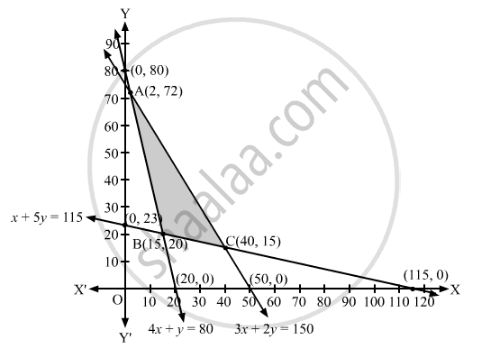# A Dietician Has to Develop a Special Diet Using Two Foods P and Q.Each Packet (Containing 30 G) of Food P Contains 12 Units of Calcium, 4 Units of Iron, 6 Units of Cholesterol and 6 Units of Vitamin a - Mathematics

Sum

A dietician has to develop a special diet using two foods P and Q. Each packet (containing 30 g) of food P contains 12 units of calcium, 4 units of iron, 6 units of cholesterol and 6 units of vitamin A. Each packet of the same quantity of food Q contains 3 units of calcium, 20 units of iron, 4 units of cholesterol and 3 units of vitamin A. The diet requires atleast 240 units of calcium, atleast 460 units of iron and at most 300 units of cholesterol. How many packets of each food should be used to minimise the amount of vitamin A in the diet? What is the minimum of vitamin A.

#### Solution

Let x packets of food P and packets of food Q be used to make the diet.

Each packet of food P contains 6 units of vitamin A and each packet of food Q contains 3 units of vitamin A. Therefore, x packets of food P and packets of food Q contains (6x+ 3y) units of vitamin A.

Since each packet of food P contains 12 units of calcium and each packet of food Q contains 3 units of calcium, therefore, x packets food P and y packets of food Q will contain (12x + 4y) units of calcium. But, the diet should contain atleast 240 units of calcium.

∴ 12x + 3≥ 240

⇒ 4x + ≥ 80

Similarly, x packets of food P and y packets of food Q will contain (4x + 20y) units of iron. But, the diet should contain atleast 460 units of iron.

∴ 4x + 20y  ≥ 460

⇒ x + 5≥ 115

Also, x packets of food P and y packets of food Q will contain (6x + 4y) units of cholesterol. But, the diet should contain atmost 300 units of cholesterol.

∴ 6x + 4y  ≤ 300

⇒ 3x + 2≤ 150

Thus, the given linear programming problem is

Minimise Z = 6x + 3y

subject to the constraints

4x + ≥ 80

x + 5≥ 115

3x + 2≤ 150

x, y ≥ 0

The feasible region determined by the given constraints can be diagrammatically represented as,The coordinates of the corner points of the feasible region are A(2, 72), B(15, 20) and C(40, 15).

The value of the objective function at these points are given in the following table.

 Corner Point Z = 6x + 3y (2, 72) 6 × 0 + 3 × 72 = 216 (15, 20) 6 × 15 + 3 × 20 = 150 (40, 15) 6 × 40 + 3 × 15 = 285

The smallest value of Z is 150 which is obtained at x = 15 and y = 20.

It can be verified that the open half-plane represented by 6x + 3y < 150 has no common points with the feasible region.

Thus, 15 packets of food P and 20 packets of food Q should be used to minimise the amount of vitamin A in the diet.

Hence, the minimum amount of vitamin A is 150 units.

Concept: Graphical Method of Solving Linear Programming Problems
Is there an error in this question or solution?

#### APPEARS IN

RD Sharma Class 12 Maths
Chapter 30 Linear programming
Exercise 30.3 | Q 12 | Page 40